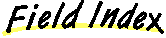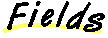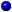`All Packages  Class Hierarchy  This Package  Previous  Next  Index`

# Class java.lang.Short

```java.lang.Object
|
+----java.lang.Number
|
+----java.lang.Short
```

public final class Short
extends Number
The Short class is the standard wrapper for short values.

Number

##MAX_VALUE
The maximum value a Short can have.MIN_VALUE
The minimum value a Short can have.TYPE
The Class object representing the primitive type short.

##Short(short)
Constructs a Short object initialized to the specified short value.Short(String)
Constructs a Short object initialized to the value specified by the String parameter.

##byteValue()
Returns the value of this Short as a byte.decode(String)
Decodes a String into a Short.doubleValue()
Returns the value of this Short as a double.equals(Object)
Compares this object to the specified object.floatValue()
Returns the value of this Short as a float.hashCode()
Returns a hashcode for this Short.intValue()
Returns the value of this Short as an int.longValue()
Returns the value of this Short as a long.parseShort(String)
Assuming the specified String represents a short, returns that short's value.parseShort(String, int)
Assuming the specified String represents a short, returns that short's value.shortValue()
Returns the value of this Short as a short.toString()
Returns a String object representing this Short's value.toString(short)
Returns a new String object representing the specified Short.valueOf(String)
Assuming the specified String represents a short, returns a new Short object initialized to that value.valueOf(String, int)
Assuming the specified String represents a short, returns a new Short object initialized to that value.

##MIN_VALUE
``` public static final short MIN_VALUE
```
The minimum value a Short can have.MAX_VALUE
``` public static final short MAX_VALUE
```
The maximum value a Short can have.TYPE
``` public static final Class TYPE
```
The Class object representing the primitive type short.

##Short
``` public Short(short value)
```
Constructs a Short object initialized to the specified short value.

Parameters:
value - the initial value of the ShortShort
``` public Short(String s) throws NumberFormatException
```
Constructs a Short object initialized to the value specified by the String parameter. The radix is assumed to be 10.

Parameters:
s - the String to be converted to a Short
Throws: NumberFormatException
If the String does not contain a parsable short.

##toString
``` public static String toString(short s)
```
Returns a new String object representing the specified Short. The radix is assumed to be 10.

Parameters:
s - the short to be convertedparseShort
``` public static short parseShort(String s) throws NumberFormatException
```
Assuming the specified String represents a short, returns that short's value. Throws an exception if the String cannot be parsed as a short. The radix is assumed to be 10.

Parameters:
s - the String containing the short
Throws: NumberFormatException
If the string does not contain a parsable short.parseShort
``` public static short parseShort(String s,
```
Assuming the specified String represents a short, returns that short's value. Throws an exception if the String cannot be parsed as a short.

Parameters:
s - the String containing the short
Throws: NumberFormatException
If the String does not contain a parsable short.valueOf
``` public static Short valueOf(String s,
```
Assuming the specified String represents a short, returns a new Short object initialized to that value. Throws an exception if the String cannot be parsed as a short.

Parameters:
s - the String containing the integer
Throws: NumberFormatException
If the String does not contain a parsable short.valueOf
``` public static Short valueOf(String s) throws NumberFormatException
```
Assuming the specified String represents a short, returns a new Short object initialized to that value. Throws an exception if the String cannot be parsed as a short.

Parameters:
s - the String containing the integer
Throws: NumberFormatException
If the String does not contain a parsable short.decode
``` public static Short decode(String nm) throws NumberFormatException
```
Decodes a String into a Short. The String may represent decimal, hexadecimal, and octal numbers.

Parameters:
nm - the string to decodebyteValue
``` public byte byteValue()
```
Returns the value of this Short as a byte.

Overrides:
byteValue in class NumbershortValue
``` public short shortValue()
```
Returns the value of this Short as a short.

Overrides:
shortValue in class NumberintValue
``` public int intValue()
```
Returns the value of this Short as an int.

Overrides:
intValue in class NumberlongValue
``` public long longValue()
```
Returns the value of this Short as a long.

Overrides:
longValue in class NumberfloatValue
``` public float floatValue()
```
Returns the value of this Short as a float.

Overrides:
floatValue in class NumberdoubleValue
``` public double doubleValue()
```
Returns the value of this Short as a double.

Overrides:
doubleValue in class NumbertoString
``` public String toString()
```
Returns a String object representing this Short's value.

Overrides:
toString in class ObjecthashCode
``` public int hashCode()
```
Returns a hashcode for this Short.

Overrides:
hashCode in class Objectequals
``` public boolean equals(Object obj)
```
Compares this object to the specified object.

Parameters:
obj - the object to compare with
Returns:
true if the objects are the same; false otherwise.
Overrides:
equals in class Object

`All Packages  Class Hierarchy  This Package  Previous  Next  Index`

Submit a bug or feature